# NMTA Math: Solving Trigonometric Equations Chapter Exam

Exam Instructions:

Choose your answers to the questions and click 'Next' to see the next set of questions. You can skip questions if you would like and come back to them later with the yellow "Go To First Skipped Question" button. When you have completed the practice exam, a green submit button will appear. Click it to see your results. Good luck!

### Page 1

#### Question 2 2. Solve the following trigonometric equation for θ.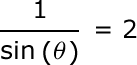### Page 3

#### Question 11 11. Solve the following trigonometric equation for x.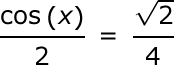### Page 4

#### Question 17 17. Solve the following equation for x.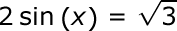#### Question 18 18. Solve the following equation for θ.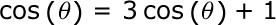#### Question 19 19. Solve for the value of x in the trigonometric equation below. (Use the domain -π/2 to π/2)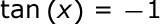### Page 5

#### NMTA Math: Solving Trigonometric Equations Chapter Exam Instructions

Choose your answers to the questions and click 'Next' to see the next set of questions. You can skip questions if you would like and come back to them later with the yellow "Go To First Skipped Question" button. When you have completed the practice exam, a green submit button will appear. Click it to see your results. Good luck!

Support